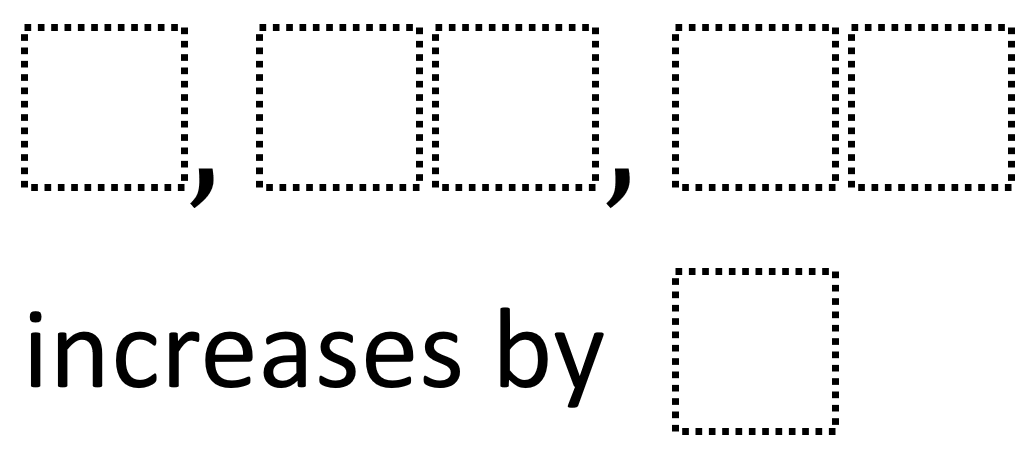# Simple Patterns 2

Directions: Using the digits 1 to 9 at most one time each, place a digit in each box to make a true pattern where the pattern increases by the smallest amount possible.### Hint

How can we tell whether the digits we place in the boxes actually make a pattern? How can we tell if the pattern increases each time by the digit we choose? How can we tell what would be a bad digit to choose for how much it increases by?

The pattern that increases by the smallest amount possible is 9, 16, 23 which increases by 7.

Source: Robert Kaplinsky

### Check Also

1.